Next: Partition functions Up: Applications of statistical thermodynamics Previous: Paramagnetism

## Mean values

Consider a system in contact with a heat reservoir. The systems in the representative ensemble are distributed over their accessible states in accordance with the Boltzmann distribution. Thus, the probability of occurrence of some statewith energyis given by(395)

The mean energy is written(396)

where the sum is taken over all states of the system, irrespective of their energy. Note that(397)

where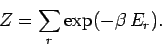(398)

It follows that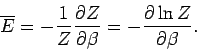(399)

The quantity, which is defined as the sum of the Boltzmann factor over all states, irrespective of their energy, is called the partition function. We have just demonstrated that it is fairly easy to work out the mean energy of a system using its partition function. In fact, as we shall discover, it is easy to calculate virtually any piece of statistical information using the partition function.

Let us evaluate the variance of the energy. We know that(400)

(see Sect. 2). Now, according to the Boltzmann distribution,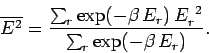(401)

However,(402)

Hence,(403)

We can also write(404)

where use has been made of Eq. (399). It follows from Eq. (400) that(405)

Thus, the variance of the energy can be worked out from the partition function almost as easily as the mean energy. Since, by definition, a variance can never be negative, it follows that, or, equivalently,. Hence, the mean energy of a system governed by the Boltzmann distribution always increases with temperature.

Suppose that the system is characterized by a single external parameter(such as its volume). The generalization to the case where there are several external parameters is obvious. Consider a quasi-static change of the external parameter fromto. In this process, the energy of the system in statechanges by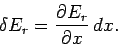(406)

The macroscopic work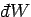done by the system due to this parameter change is(407)

In other words, the work done is minus the average change in internal energy of the system, where the average is calculated using the Boltzmann distribution. We can write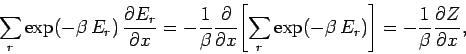(408)

which gives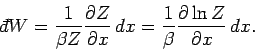(409)

We also have the following general expression for the work done by the system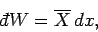(410)

where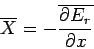(411)

is the mean generalized force conjugate to(see Sect. 4). It follows that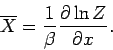(412)

Suppose that the external parameter is the volume, so. It follows that(413)

and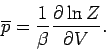(414)

Since the partition function is a function of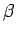and(the energiesdepend on), it is clear that the above equation relates the mean pressure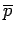to(via) and. In other words, the above expression is the equation of state. Hence, we can work out the pressure, and even the equation of state, using the partition function.Next: Partition functions Up: Applications of statistical thermodynamics Previous: Paramagnetism
Richard Fitzpatrick 2006-02-02